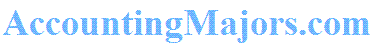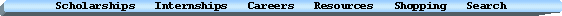# Interest Rate Hedges - Strategies for Swaps & Derivatives

Click Here for a simple guide (PDF) on Hedging Interest Rate Risk with Swaps & Derivatives

Learn about the differences between a fair value hedge and a cash flow hedge, and which is more appropriate based upon interest rate expectations. Learn how either assets or liabilities can be hedged, using swaps or other derivatives, such as caps or floors. The interest rate hedge guide, illustrates the concepts detailed in FASB's accounting standard for derivatives, SFAS 133, as ammended by SFAS 138, 149, and the various DIG issues.